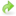# First steps¶

## Foreword¶

If you have never used the FEniCS system before, you should first read their tutorial. If you’re not familiar with adjoints and their uses, see the background.

## A first example¶

Let’s suppose you are interested in solving the nonlinear time-dependent Burgers equation:

$\frac{\partial \vec u}{\partial t} - \nu \nabla^2 \vec u + \vec u \cdot \nabla \vec u = 0,$

subject to some initial and boundary conditions.

A forward model that solves this problem with P2 finite elements might look as follows:

from fenics import *

n = 30
mesh = UnitSquareMesh(n, n)
V = VectorFunctionSpace(mesh, "CG", 2)

u = project(Expression(("sin(2*pi*x)", "cos(2*pi*x)"), degree=2),  V)

u_next = Function(V)
v = TestFunction(V)

nu = Constant(0.0001)

timestep = Constant(0.01)

F = (inner((u_next - u)/timestep, v)

bc = DirichletBC(V, (0.0, 0.0), "on_boundary")

t = 0.0
end = 0.1
while (t <= end):
solve(F == 0, u_next, bc)
u.assign(u_next)
t += float(timestep)You can download the source code and follow along as we adjoin this code.

from fenics import *


The reason why it is necessary to do it afterwards is because dolfin-adjoint overloads many of the dolfin API functions to understand what the forward code is doing. In this particular case, the solve function and assign method have been overloaded:

 while (t <= end):
solve(F == 0, u_next, bc)
u.assign(u_next)


The dolfin-adjoint versions of these functions will record each step of the model, building an annotation, so that it can symbolically manipulate the recorded equations to derive the tangent linear and adjoint models. Note that no user code had to be changed: it happens fully automatically.

In order to talk about adjoints, one needs to consider a particular functional. While dolfin-adjoint supports arbitrary functionals, let us consider a simple nonlinear example. Suppose our functional of interest is the square of the norm of the final velocity:

$J(u) = \int_{\Omega} \left\langle u(T), u(T) \right\rangle \ \textrm{d}\Omega,$

or in code:

J = assemble(inner(u, u)*dx),


where u is the final velocity.

Suppose we wish to compute the gradient of $$J$$ with respect to the initial condition for $$u$$, using the adjoint. Then since u is updated over time we must tell dolfin-adjoint that we are interested in the initial value of :py:datau by adding:

control = Control(u)


after initializing u. We can then add the following code to compute the gradient:

dJdu = compute_gradient(J, control)


This single function call differentiates the model, assembles each adjoint equation in turn, and then uses the adjoint solutions to compute the requested gradient.

If we wish instead to take the gradient with respect to the diffusivity $$\nu$$, we can write:

dJdnu = compute_gradient(J, Control(nu))


If we want both gradients we can write

dJdu, dJdnu = compute_gradient(J, [control, Control(nu)])


Now our whole program is

from fenics import *

n = 30
mesh = UnitSquareMesh(n, n)
V = VectorFunctionSpace(mesh, "CG", 2)

u = project(Expression(("sin(2*pi*x)", "cos(2*pi*x)"), degree=2),  V)
control = Control(u)

u_next = Function(V)
v = TestFunction(V)

nu = Constant(0.0001)

timestep = Constant(0.01)

F = (inner((u_next - u)/timestep, v)

bc = DirichletBC(V, (0.0, 0.0), "on_boundary")

t = 0.0
end = 0.1
while (t <= end):
solve(F == 0, u_next, bc)
u.assign(u_next)
t += float(timestep)

J = assemble(inner(u, u)*dx)
dJdu, dJdnu = compute_gradient(J, [control, Control(nu)])


Observe how the changes required from the original forward code to the adjoined version are very small: with only three lines added to the original code, we are able to compute the gradient information.If you have been following along, you can download the adjoined Burgers’ equation code and compare your results.

Once you have computed the gradient, how do you know if it is correct?

dolfin-adjoint offers easy routines to rigorously verify the computed results, which is the topic of the next section.